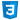# The CSS calc() function

The CSS `calc()` function allows you to apply basic math operations on length values:

• Add `+`
• Subtract `-`
• Multiply `*`
• Division `/`

For example let’s say that you want to use a percentage width of 100% on an element, but you want to reserve a total of 32px width on that same element. Here’s how to do that with `calc()`:

``````div {
max-width: calc(100% - 32px);
}``````

Now that element will take up 100% of whatever container it sits inside, minus 32px.

Result:

This is useful whenever you need to work with exact percentage and pixel amounts at the same time on a UI.

You can also easily center align the element inside its container:

``````div {
max-width: calc(100% - 32px);
margin-left: auto;
margin-right: auto;
}``````

Result:

Now the center-aligned element has exactly 16px of spacing on the left and the right. As you can tell, you can use this `calc()` as a replacement for using padding or margin when you add spacing between your elements.